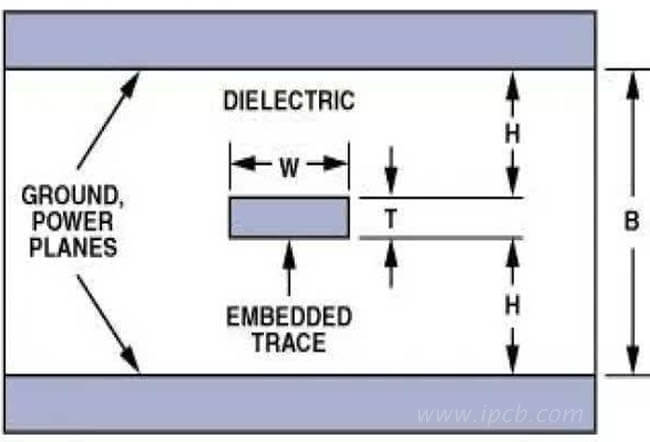전문 고주파 회로 기 판,고속 회로 기 판,IC 패 키 징 기 판,반도체 테스트 판,HDI 회로 기 판,소프트 하 드 결합 판,양면 다 층 판,PCB 디자인 및 PCBA 제조

### 빠 른 가격 획득.

sales@ipcb.com# PCB기술## PCB기술

PCB characteristic impedance control technology

1. Definition of signal transmission line

(1) According to the principle of electromagnetic wave, the shorter the wavelength (λ), the higher the frequency (f). The product of the two is the speed of light. That is, C = λ. F = 3 × 1010 cm / s

(2) Although any component has a high signal transmission frequency, the original high transmission frequency will be reduced or the time will be delayed after transmission through PCB wire.

Therefore, the shorter the wire length, the better.

(3) It is advantageous to increase the PCB wiring density or shorten the wire size. However, with the acceleration of component frequency or the shortening of pulse period, the wire length is close to a certain range of signal wavelength (speed). At this time, when the component is transmitted in PCB wire, obvious "distortion" will appear.

(4) 3.4.4 of ipc-2141 points out that when the signal is transmitted in the wire, if the length of the wire is close to 1 / 7 of the signal wavelength, then the wire is regarded as the signal transmission line.

(5) For example:

If the signal transmission frequency (f) of a component is 10MHz and the wire length on PCB is 50cm, should characteristic impedance control be considered?

Solution: C = λ. F = 3 × 1010 cm / s

λ＝C/f＝（3 ×1010 cm/s）/（1 ×107 /s ）＝3000cm

Wire length / signal wavelength = 50 / 3000 = 1 / 60

Because: 1 / 60 "1 / 7", so this conductor is a common conductor, so the characteristic impedance does not need to be considered.

In electromagnetic wave theory, Maxwell formula tells us that the propagation velocity vs of sine wave signal in medium is directly proportional to the speed of light C, but inversely proportional to the dielectric constant of transmission medium.

VS ＝C/√εr

When ε r = 1, the signal transmission reaches the speed of light propagation, i.e. 3 × 1010 cm / s.PCB characteristic impedance

2. Transmission rate and dielectric constant

Signal transmission speed of different plates at 30MHz

Transmission speed of dielectric constant signal (M / μ s) of dielectric material TG (° C)

Vacuum / 1.0 300.00

PTFE / 2.202.26

Thermosetting polypropylene ether 210 2.5189.74

Cyanate ester resin 225 3.0 173.21

Polytetrafluoroethylene resin + E glass cloth / 2.6186.25

Cyanate ester resin + glass cloth 225 3.7155.96

Polyimide + glass cloth 230 4.5141.42

Quartz / 3.9 151.98

Epoxy resin glass cloth 130 ± 54.7138.38

Aluminum / 9.0 100.00

It can be seen from the above table that with the increase of dielectric constant (ε R), the transmission speed of signal in dielectric material decreases. In order to obtain high signal transmission speed, it is necessary to adopt high characteristic impedance value; for high characteristic impedance, low dielectric constant (ε R) material must be selected; and the dielectric constant (ε R) of Teflon should be fast.

FR-4 plate is composed of epoxy resin and E-grade glass cloth, and its dielectric constant (ε R) is 4.7. The signal transmission speed is 138m / μ s. The dielectric constant (ε R) can be easily changed by changing the resin system.